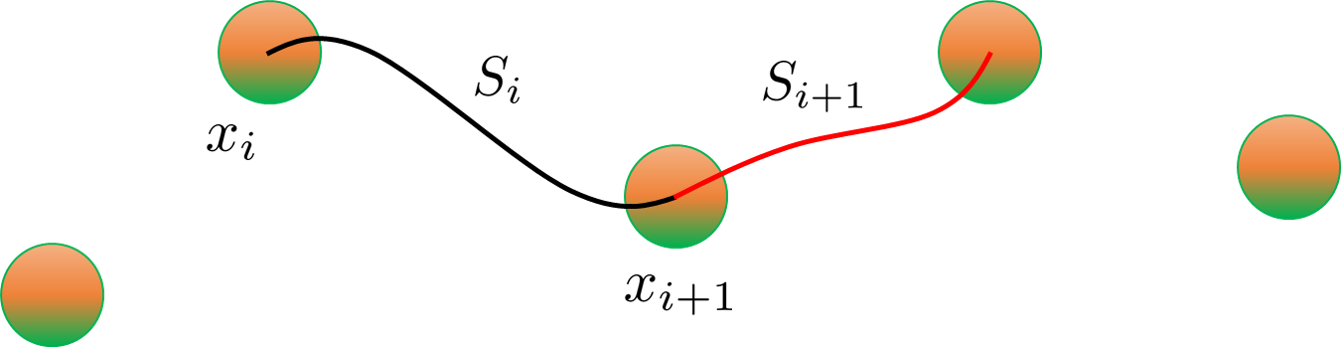# Joint Trajectory Interpolation

## Interpolation with Cubic Spline

IndySDK allows users to modify trajectory generation methods as well as low-level controllers. This example describes how to develop joint trajectory interpolators with cubic splines. More specifically, the interpolator will be designed to arrive at the target point after a certain time.

To this end, it is required to find the coefficients of the $S_{i}$ function to satisfy the following four conditions.• $S_{j}(x_{j}) = f(x_{j})$, $S_{j}(x_{j+1})=f(x_{j+1})$, $\forall$ $j=0,1,\cdots n-1$
• $S_{j+1}(x_{j+1}) = S_{j}(x_{j+1})$, $\forall$ $j=0,1,\cdots n-2$
• $S_{j+1}^{'}(x_{j+1}) = S_{j}^{'}(x_{j+1})$, $\forall$ $j=0,1,\cdots n-2$
• $S_{j+1}^{''}(x_{j+1}) = S_{j}^{''}(x_{j+1})$, $\forall$ $j=0,1,\cdots n-2$

Using one of the two conditions given below, the coefficient for a cubic spline can be calculated.

• $S^{''}(x_{0}) = S^{''}(x_{n}) = 0$
• $S^{'}(x_{0}) = f^{'}(x_{0})$, $S^{'}(x_{n}) = f^{'}(x_{n})$

## Example Code

As a first step, generate the joint trajectory interpolation component template. Then, add several functions and variables to the header like follows:

  1 2 3 4 5 6 7 8 9 10 11 12 13 14 15 16 17 18 19 20 21 22 //interpolate paths void Interpolate(); // coefficients: q(s) = a0*s^3 + a1*s^2 + a2*s + a3 Eigen::MatrixXd a0; Eigen::MatrixXd a1; Eigen::MatrixXd a2; Eigen::MatrixXd a3; double _t0; double _s; double _nSegment; double _segTime; VecType _readyPos; bool _isTargetReached = false; bool _isTrajSet = false; std::vector _Waypoint; int nPts; int nSplines; int dim; 

Joint trajectory interpolation component consists of setPath and traj functions. First, setPath is used to set the path with waypoints defined by the user interface (e.g., Conty). traj generates the trajectory to be used for the controller at every sampling time.

Fill in the setPath function based on the waypoints given in the form of vector-type pointer:

  1 2 3 4 5 6 7 8 9 10 11 12 13 14 15 16 17 18 19 20 21 22 23 24 25 26 27 28 29 30 31 void JointTrajectoryInterpolationComponent::setPath(const VecType *path, int len, double * maxDeviation) { std::vector wp_angle; _readyPos = path; wp_angle.push_back(path); for (int i = 1; i< len; i++) { wp_angle.push_back(path[i]); } _Waypoint = wp_angle; nPts = wp_angle.size(); nSplines = wp_angle.size()-1; dim = 6; // interpolation a0.resize(dim, nSplines); a1.resize(dim, nSplines); a2.resize(dim, nSplines); a3.resize(dim, nSplines); Interpolate(); _nSegment = len-1; printf("set Path\n"); } 

This example uses a cubic spline to set the path between waypoints: The coefficients that satisfy above-mentioned conditions can be calculated by constructing a specific matrix given as follow (Each bundle of code indicated by comments represents which part describes which condition):

  1 2 3 4 5 6 7 8 9 10 11 12 13 14 15 16 17 18 19 20 21 22 23 24 25 26 27 28 29 30 31 32 33 34 35 36 37 38 39 40 41 42 43 44 45 46 47 48 49 50 51 52 53 54 55 56 57 58 59 60 61 void JointTrajectoryInterpolationComponent::Interpolate() { Eigen::MatrixXd coeff_A; Eigen::MatrixXd coeff_b; Eigen::MatrixXd coeff; for (int j=0; j

Next, define the parameters to generate the trajectory in setTraj function. In this example, the time required to move between waypoints is set to 10 seconds.

 1 2 3 4 5 6 7 8 void JointTrajectoryInterpolationComponent::setTraj(double t0) { _t0 = t0; _segTime = 10.0; _isTargetReached = false; _isTrajSet = true; } 

Now, fill in the traj function to generate real-time trajectory. Based on the coefficients calculated from Interpolate() function, desired position, desired velocity, and desired acceleration are calculated. Note that this example uses a linear function with a slope of 1/10 because arbitrary integers (i.e., s=0, 1, 2, ...) were used for waypoints when generating the path.

  1 2 3 4 5 6 7 8 9 10 11 12 13 14 15 16 17 18 19 20 21 22 23 24 25 26 27 28 29 30 31 32 33 34 35 36 37 38 39 40 41 42 43 44 45 46 void JointTrajectoryInterpolationComponent::traj(double time, VecType & posDes, VecType & velDes, VecType & accDes) { if (time <= _t0) { posDes = _readyPos; velDes = VecType::Zero(); accDes = VecType::Zero(); return; } _s = (time-_t0)/_segTime; double sdot = 1.0/_segTime; double sddot = 0.0; if (_s > nSplines) _s = nSplines; int idx; if (_s < nSplines) { idx = (int)floor(_s); }else { idx= nSplines-1; } for (int i=0; i< dim; i++) { posDes[i] = a0(i, idx)*pow(_s, 3) + a1(i, idx)*pow(_s, 2) + a2(i, idx)*_s + a3(i, idx); velDes[i] = (3.0*a0(i, idx)*pow(_s, 2) + 2.0*a1(i, idx)*_s + a2(i, idx))*sdot; accDes[i] = (3.0*a0(i, idx)*pow(_s, 2) + 2.0*a1(i, idx)*_s + a2(i, idx))*sddot + (6.0*a0(i, idx)*_s + 2.0*a1(i, idx))*sdot*sdot; } if (_t0 + _nSegment*_segTime <= time) { _readyPos = posDes; _isTrajSet = false; _isTargetReached = true; _s = 0.0; } } 

Finally, the following functions are used so that the controller can bring the parameters from the interpolator.

• setInitialTime brings the control time to define the starting time of the trajectory.
 1 2 3 4 void JointTrajectoryInterpolationComponent::setInitialTime(double t0) { _t0 = t0; } 
• getDuration allows the controller to get the time required to finish the generated trajectory.
 1 2 3 4 double JointTrajectoryInterpolationComponent::getDuration() { return _nSegment*_segTime; } 
• getNTotalSegment allows the controller to call the number of waypoints.
 1 2 3 4 int JointTrajectoryInterpolationComponent::getNTotalSegment() { return _nSegment; } 
• isTargetReached allows the controller to check whether the robot arrives at the target point.
 1 2 3 4 bool JointTrajectoryInterpolationComponent::isTargetReached() { return _isTargetReached; }